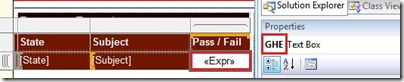# Display Current Page Total in Footer

There is one interesting story about NASA floating around the internet for quite some time now. The story goes like this – NASA spent millions to develop a pen that could write in space under zero gravity while the Soviet cosmonauts just used a pencil. Even though this is just a myth, I found it pretty interesting as sometimes even I tend to overdo things and come up with complicated answers when simple solutions exist. One of my latest blunders was on how to display current page totals of a multi-paged report in the footer.For demonstrating the solution, I have made a dataset which will return a 1 or 0 based on whether all the students of a state has passed for a particular subject. Since the number of rows returned would be pretty large, the report will span over multiple pages. The requirement is to display the total number of pass and fails of the current page in the report footer. The steps are given below:- (I) Overkill Solution Thinking there is no straight forward way of doing this, I tried to devise the solution by applying the concepts of limiting rows in a page and custom code. 1) In the design mode of BIDS, create a matrix with State, Sector and the Pass/Fail measure. Then use the technique detailed in this blog by Melissa Coates to limit rows on a page.The expression that I have used for this demo is =Ceiling(Rownumber(Nothing)/12) as I need to limit 12 rows in my page. This can be modified as per your requirements. 2) Click on Report on the top menu and then select Report Properties. Navigate to the Code section and paste the custom code given below Dim public Sales as Integer
Dim public Shared FirstMember as Integer
Dim public Cnt as Integer=0 Public Function FindFirst(ByVal Sales AS Integer ) AS Integer
Cnt=Cnt+1
if (Cnt mod 12) = 1 then
FirstMember=Sales
Else
FirstMember=FirstMember+Sales
End If
return Sales
End Function This code will sum up the values which are passed as input to the function and keep the result in FirstMember variable. Since we know that only 12 rows will be displayed in one page, a counter variable is used to reset the value of FirstMember once the 13th row or multiples of it are reached. Thus it is ensured that the sum of the values in each page can be obtained by accessing the FirstMember variable. 3) Edit the measure expression in the matrix and paste the following code there =Code.FindFirst(Sum(Fields!GHE.Value)) This expression will invoke the function and pass the measure value each time a row is displayed.GHE is the name of my measure and Fields!GHE.Value should be substituted by your measure name. 4) Now you can directly use the following expressions in a textbox in your footer to get the number of pass and fail in each page. =”No of Pass in current Page =” & Code.FirstMember =”No of Fail in current Page =” & 12-Code.FirstMember If the number of rows that you are using is something other than 12, then it should be substituted accordingly in the expression for number of fails.(II) Simple Solution 1) Make a simple matrix with the required columns and measure (Here, the columns are State and Sector & the measure is GHE) 2) Paste the following expression in a textbox in the footer =”No of Pass in current Page =” & Sum(ReportItems(“GHE”).Value) =”No of Pass in current Page =” & Sum(iif(ReportItems(“GHE”).Value=0,1,0)) The GHE in ReportItems(“GHE”).Value is the name of the textbox in which the measure is placed. The name of the textbox can be got by right clicking on the textbox and then pressing F4.3) Now the report can be previewed and the same result can be viewed. Moral of the blog is to make sure that you do your research properly and then get it reviewed by your peers before coming up with complex solutions.### Posted by SQLJason